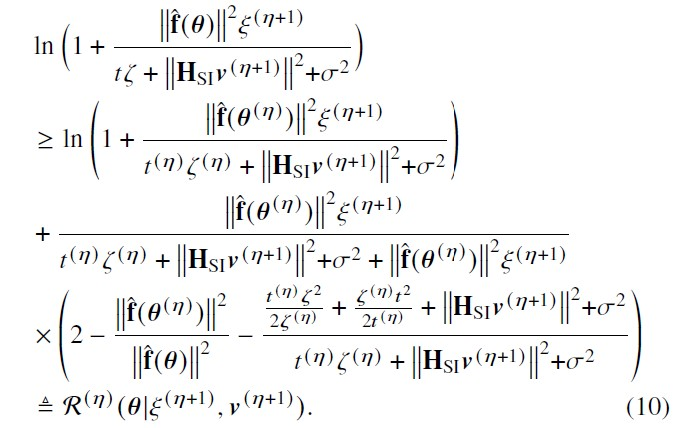Yes Sir. Thanks for the valuable suggestions regarding the usage of the tool.

This is the code:
obj_4 = log ( 1 + ( (f_theta’f_theta)P_UE_inc) / ( t_initialx_initial …
+ square_pos(norm(h_s_i
Tx_beamforming_inc)) + sigma_sqr ) );
obj_5 = ( (f_theta’f_theta)P_UE_inc) / ( t_initialx_initial + …
square_pos(norm(h_s_i
Tx_beamforming_inc)) + sigma_sqr + (f_theta’*f_theta)*P_UE_inc ) ;

``````       obj_6 = ( ( (t_initial*square_pos(x))/(2*x_initial) ) + ((x_initial*square_pos(t))/(2*t_initial)) ...
+ (square_pos(norm(h_s_i*Tx_beamforming_inc))) + (sigma_sqr) ) / (t_initial*x_initial ...
+ square_pos(norm(h_s_i*Tx_beamforming_inc))  + sigma_sqr  )  ;

obj_7 = obj_5*( 2 - (f_theta'*f_theta).*inv_pos(square_pos(norm(f_theta_opt))) - obj_6) ;

obj_8 =  obj_4 + obj_7; % Equation (10)
``````

The equation for the above code is shown below:Getting Error:

Error using cvx/pow_cvx
Disciplined convex programming error:
Illegal operation: pow_p( {convex}, {-1} )

Error in cvx/inv_pos (line 5)
y = pow_cvx( x, -1, ‘pow_p’ );

Error in convergence_beam_main_1 (line 319)
obj_7 = obj_5*( 2 - (f_theta’*f_theta).*inv_pos(square_pos(norm(f_theta_opt))) - obj_6) ;

Basically, the objective function is non-concave (Verified) only.
Reason: Term1 and Term2 is constant. Term 3: (2 - (constant/convex) - convex) = 2-concave-convex = concave only.

But, I am getting error in writing that last part of Term 3.
Please help me in writing that part-3 : Even though the entire term is concave, unable to write according to the syntax of the cvx tool.
As it is not accepting ( inv_pos(Convex) ), but how to write 1/convex?
inv_pos only accepts concave (or affine).

The reciprocal of a convex function, even if positive everywhere, is not necessarily concave or convex, and might change between concave and convex at different argument values. Hence, CVX can’t deal with such functions in general, even if they are restricted to be positive. `1/norm([x;1])` is a simple example of such a function which changes between concave and convex at different argument values; hence is not representable in CVX.

Sir, My function is 1/norm(f_theta)^2;
Where f_theta = f + a_u*diag(h)*phase_shift; Here, phase_shift is the optimization variable.

And cvx is accepting following command:

inv_pos(square_pos(norm(f_theta_opt))).

Inserting a minus sign on the argument of `inv_pos` turns a convex argument into a concave argument; hence CVX accepts it. But is is of course a DIFFERENT and very much not equivalent model. And `inv_pos` of an argument which is guaranteed to be <= 0 is just complete garbage, even though CVX wlll accept it.

If you wanted to maximize x^2, and because CVX wouldn’t accept it, you changed it to maximize -x^2, would you view that as a legitimate way of solving your problem? Of course it wouldn’t be. The same applies in your situation.

Please step back, and carefully reexamine your problem. Prove that your problem formulation is a convex optimization problem. Otherwise CVX isn’t the right tool for it.

Sir, the command inv_pos(-square_pos(norm(f_theta_opt))) is accepting but not giving proper values also’

I have verified that the problem is concave only. Hence trying to maximize the objective function.

That’s why I asked you the following question:

But, I am getting error in writing that last part of Term 3.
Please help me in writing that part-3 : Even though the entire term is concave, unable to write according to the syntax of the cvx tool.
As it is not accepting ( inv_pos(Convex) ), but how to write 1/convex?
inv_pos only accepts concave (or affine).

Please re-read mt previous post. And this time take it to heart.

According to your suggestions , above objective function can not be represented by the cvx Tool.

I thought that another tool may be used to solve??

YALMIP can handle DCP formulations, as well as non-convex and non-DCP convex formulations.